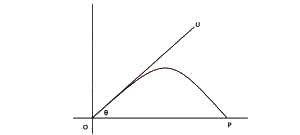# The diagram above represents the trajectory of a projectile with an initial velocity U. Calculate the time taken to reach the maximum height. (Take g as acc...

Question 1

The diagram above represents the trajectory of a projectile with an initial velocity U. Calculate the time taken to reach the maximum height. (Take g as acceleration due to gravity)